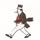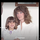## Social Question#### How would you do this simple calculation?

Asked by LostInParadise (29640) May 22nd, 2020
5 responses
“Great Question” (1)

The lights went out the other night. If you have an analog clock and a digital clock, this allows you to determine when the lights went out and when they came back on. In this case, the analog clock was almost identical to my watch, so the lights were not out for very long. To make this problem more interesting, I will make up some numbers.

Suppose that the digital clock reads 3:30, the analog clock reads 6:30, and the watch is at 7:00. From the digital clock, we know the blackout ended 3½ hours ago, so the lights went on at 3:30. When did the lights go out?

I am not interested in the answer so much as the method you use to find it. My initial way of solving the problem was much more complicated than it needed to be. I want to see if you can find the really easy method of determining the answer, which I found to be somewhat counterintuitive, for reasons that I will give later. Be warned that I have presented the problem so as to encourage you to use the same faulty method I used.Follow this questionSend to a friend!Topics: , ,
Observing members: 0Composing members: 0The digital clock started up 3 and ½ hours ago. Since the watch reads 7, the power failed at 3:30.

stanleybmanly (24138)“Great Answer” (3)3:30 is when the power went back on.

LostInParadise (29640)“Great Answer” (0)I think I understand now. The analog clock is 30 minutes behind the watch. Which means that the power outage lasted 30 minutes. If both clocks stopped then resumed power simultaneously at 3:30, the lights went out at 3.

stanleybmanly (24138)“Great Answer” (1)That is correct and that is how I initially solved the problem, but there is a simpler solution.

Let’s look at it algebraically. Let TD be the digital time, TA be the analog time and T be the correct time. The lights went on at T – TD. The duration is T – TA, so the lights went out at T-TD – (T- TA) = TA – TD.

This seemed very odd, because it says that we do not need to know the actual time to figure out when the lights went out, but it does make sense. TA – TD is what the analog clock read when the lights went on. But this is the same thing that it read when the lights went out, so we have 6:30 – 3½ hours, giving 3:00

LostInParadise (29640)“Great Answer” (1)What time did the lights go out in Georgia?

Dutchess_lll (8733)“Great Answer” (0)